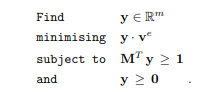# Minimising y*v^e

Hello,
My optimization problem is here:
Find y ∈ R^m
minimising `y. v^e`
subject to` M^Ty>=1 and y>=0`And my code is here but I could not write the second line(minimising y*v ^e):

cvx_begin
variable y
minimize(y * v’)
subject to
m’ * y >=1
y>=0
cvx_end

Bests,

Perhaps you’d care to tell us what `v^e` is? What are `v` and `e`? Why do you say y ∈ R^m but declare `y` as a scalar` variable? Please ensure after everything is defined, that the objective function evaluatea to a scalar.

v^e is a vector. Sorry, this is a bad name. It seems it has 2 different value e and v but actually it is “vector of emprical model” so it is a vector and M is a matrix

Write out the MATLAB code to assign values to all the input data to the CVX program. I still have no idea what problem you are trying to solve.